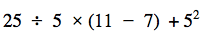Exponents
Multiplying Polynomials
Solve these
Riddles
100

(2x + 2) + (3x - 1)

5x - 1

100

Simplify:

56 x 514

520

100

What do you do when your multiplying polynomials?

Take each term of one polynomials and multiplying it by each term of the other.

100

Freebie

100

The more you have of it, the less you see

Darkness

200

subtract the polynomials

(3x2 + 4x - 5) - (4x2 - 5 + 3x)

-x2 + x

200

72

200

solve.

-3m(3m2+9)

-9m3-27m

20045

200

I come from a mine and get surrounded by wood. Everyone uses me, what am I?

A PENCIL

300

(3x4 + 6x2 + 14x - 5) + (3x3 + 3x2 - 10)

3x4 + 3x3 + 9x2 + 14x - 15

300

Simplify (129)10

1290

300

You do this when multiplying powers with the same base

keep the base the same  and add the exponents

300

5x - 14 = 8x + 4

x=-6
300

A bus driver goes the wrong way on a one way street. He passes the cops but they don't stop him. Why?

Because he was walking

400

(-3x3 + x + 3x3 + x) + (2x2 - 2x + 15)

2x2 + 15

400

What is exponent written with negative exponents 1/57

5-7

400

Solve the problem (5x+3) by (2x2+10x-6)

10x3+56x2-18

400

x + 10 +6(x+3x) +4

11x+14

400

A bus driver goes the wrong way on a one way street. He passes the cops but they don't stop him. Why?

Doors

500

Subtract the polynomials

(3x - 3x2 - 3x3 - 3x4) - (3x - 3x2 - 3x3 - 3x4)

0

500

What is the value of:

23 + 32 - 42

1

500

Multiply

(x-1)(x+4)(x+5)

x3+8x2+11x-20

500

5n + 34 = -2(1-7n)

n=4

500

What word is always pronounced wrong?

WRONG

Click to zoom